# Euler Angles To Direction Cosines VI

## LabVIEW 2018 Help

Edition Date: March 2018
Part Number: 371361R-01
View Product Info

### DOWNLOAD (Windows Only)LabVIEW 2016 HelpLabVIEW 2017 HelpLabVIEW 2018 HelpLabVIEW 2019 HelpLabVIEW 2020 Help

Owning Palette: Geometry VIs

Requires: Full Development System

Converts Euler angles into a 3-by-3 matrix of direction cosines. The VI accepts both proper and Tait-Bryan angle types.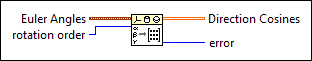Euler Angles specifies the Euler angles in radians.phi specifies the first rotation angle about the first axis in radians.theta specifies the rotation angle about the second axis in radians.psi specifies the second rotation angle about the third axis in radians.rotation order specifies the order of the axes to rotate the coordinates around. For example, X-Y-Z specifies the first, second, and third rotations are about the x-, y-, and z-axes respectively. Z-X-Z is the default order.

 0 X-Y-Z 1 X-Z-Y 2 Y-X-Z 3 Y-Z-X 4 Z-X-Y 5 Z-Y-X 6 X-Y-X 7 X-Z-X 8 Y-X-Y 9 Y-Z-Y 10 Z-X-Z 11 Z-Y-Z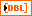Direction Cosines returns the 3-by-3 direction cosine matrix, which maps points in the old coordinate frame to points in the new coordinate frame. Each element in Direction Cosines must be in the range of [-1, 1].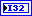error returns any error or warning from the VI. You can wire error to the Error Cluster From Error Code VI to convert the error code or warning into an error cluster.

## Euler Angles To Direction Cosines Details

You can express a rotation using direction cosines or Euler angles. The following equation describes the relationship between Euler Angles and Direction Cosines (assume the rotation is the default Z-X-Z order):

R =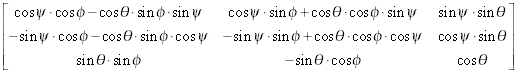where R is the output 3-by-3 Direction Cosines matrix.(–<),(0), and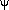(–<) are the input Euler Angles in radians.

Not Helpful